International
Tables for
Crystallography
Volume F
Crystallography of biological macromolecules
Edited by M. G. Rossmann and E. Arnold

International Tables for Crystallography (2006). Vol. F, ch. 6.1, pp. 126-127   | 1 | 2 |

U. W. Arndta

aLaboratory of Molecular Biology, Medical Research Council, Hills Road, Cambridge CB2 2QH, England

| top | pdf |

Charged particles with energy E and mass m moving in a circular orbit of radius R at a constant speed ν radiate a power, P, into a solid angle of 4π, where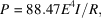where E is in GeV, I is the circulating electron or positron current in ampères and R is in metres. Thus, for example, in a bending-magnet beam line at the ESRF, Grenoble, France, R = 20 m, and at 5 GeV and 200 mA, P = 554 kW.

For relativistic electrons, the electromagnetic radiation is compressed into a fan-shaped beam tangential to the orbit, with a vertical opening angle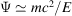, i.e. 0.1 mrad for E = 5 GeV (Fig. 6.1.2.2). This fan rotates with the circulating electrons; if the ring is filled with n bunches of electrons, a stationary observer will see n flashes of radiation every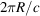s, the duration of each flash being less than 1 ns.Figure 6.1.2.2 | top | pdf |Synchrotron radiation emitted by a relativistic electron travelling in a curved trajectory. B is the magnetic field perpendicular to the plane of the electron orbit; ψ is the natural opening angle in the vertical plane; P is the direction of polarization. The slit, S, defines the length of the arc of angle, Δθ, from which the radiation is taken. From Buras & Tazzari (1984).

The spectral distribution of synchrotron radiation extends from the infrared to the X-ray region; Schwinger (1949) gives the instantaneous power radiated by a monoenergetic electron in a circular motion per unit wavelength interval as a function of wavelength (Winick, 1980). An important parameter specifying the distribution is the critical wavelength, λc: half the total power radiated, but only ∼9% of the total number of photons, is at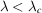(Fig. 6.1.2.6). λc (in Å) is given by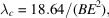where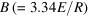is the magnetic bending field in T, E is in GeV and R is in metres.

The orbit of the particle can be maintained only if the energy lost, in the form of electromagnetic radiation, is constantly replenished. In an electron synchrotron or in a storage ring, the circulating particles are electrons or positrons maintained in a closed orbit by a magnetic field; their energy is supplied or restored by means of an oscillating radiofrequency (RF) electric field at one or more places in the orbit. In a synchrotron designed for nuclear-physics experiments, the circulating particles are injected from a linear accelerator, accelerated up to full energy by the RF field, and then deflected onto a target with a cycle frequency of about 50 Hz. The synchrotron radiation is thus produced in the form of pulses of this frequency. A storage ring, on the other hand, is filled with electrons or positrons, and after acceleration the particle energy is maintained by the RF field; ideally, the current circulates for many hours and decays only as a result of collisions with remaining gas molecules. At present, only storage rings are used as sources of synchrotron radiation, and many of these are dedicated entirely to the production of radiation: they are not used at all, or are used only for limited periods, for nuclear-physics collision experiments.

Synchrotron radiation is highly polarized. In an ideal ring, where all electrons are parallel to one another in a central orbit, the radiation in the orbital plane is linearly polarized with the electric vector lying in this plane. Outside the plane, the radiation is elliptically polarized.

In practice, the electron path in a storage ring is not a circle. The `ring' consists of an alternation of straight sections and bending magnets. Beam lines are installed at the bending magnets and at the insertion devices.

These insertion devices with a zero magnetic field integral, i.e. wigglers and undulators, may be inserted in the straight sections (Fig. 6.1.2.4). A wiggler consists of one or more dipole magnets with alternating magnetic field directions aligned transverse to the orbit. The critical wavelength can thus be shifted towards shorter values because the bending radius can be decreased over a short section, especially when superconducting magnets are used. Such a device is called a wavelength shifter. If it has N dipoles, the radiation from the different poles is added to give an N-fold increase in intensity. Wigglers can be horizontal or vertical. In a wiggler, the maximum divergence, 2α, of the electron beam is much larger than ψ, the vertical aperture of the radiation cone in the spectral region of interest (Fig. 6.1.2.2). If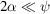, and if, in addition, the magnet poles of a multipole device have a short period, then the device becomes an undulator; interference takes place between the radiation of wavelength λ0 emitted at two points λ0 apart on the electron trajectory (Fig. 6.1.2.5). The spectrum at an angle θ to the axis observed through a pinhole consists of a single spectral line and its harmonics with wavelengths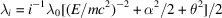(Hofmann, 1978). Typically, the bandwidth of the lines,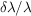, is ∼0.01 to 0.1, and the photon flux per unit bandwidth from the undulator is many orders of magnitude greater than that from a bending magnet. Undulators at the ESRF have a fundamental wavelength of less than 1 Å.Figure 6.1.2.3 | top | pdf |Comparison of the spectra from the storage ring SPEAR in photons s−1 mA−1 mrad−1 per 1% band pass (1978 performance) and a rotating-anode X-ray generator, showing the Cu K emission line and the Bremsstrahlung. Reproduced with permission from Nagel (1980). Copyright (1980) New York Academy of Sciences.Figure 6.1.2.4 | top | pdf |Main components of a dedicated electron storage-ring synchrotron-radiation source. For clarity, only one bending magnet is shown. From Buras & Tazzari (1984).Figure 6.1.2.5 | top | pdf |Electron trajectory within a multipole wiggler or undulator.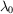is the spatial period, α is the maximum deflection angle and θ is the observation angle. From Buras & Tazzari (1984).

The spectra for a bending magnet and a wiggler are compared with that from a copper-target rotating-anode tube in Fig. 6.1.2.3.Figure 6.1.2.6 | top | pdf |Spectral distribution and critical wavelengths for (a) a dipole magnet, (b) a wavelength shifter and (c) a multipole wiggler at the ESRF. From Buras & Tazzari (1984).

### References

Hofmann, A. (1978). Quasi-monochromatic synchrotron radiation from undulators. Nucl. Instrum. Methods, 152, 17–21.Google Scholar
Schwinger, J. (1949). On the classical radiation of accelerated electrons. Phys. Rev. 75, 1912–1925.Google Scholar
Winick, H. (1980). Properties of synchrotron radiation. In Synchrotron radiation research, edited by H. Winick & S. Doniach. New York: Plenum.Google Scholar